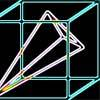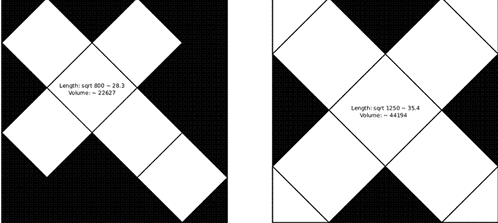#### You may also likeA box of size a cm by b cm by c cm is to be wrapped with a square piece of wrapping paper. Without cutting the paper what is the smallest square this can be?### Plane to See

P is the midpoint of an edge of a cube and Q divides another edge in the ratio 1 to 4. Find the ratio of the volumes of the two pieces of the cube cut by a plane through PQ and a vertex.### Four Points on a Cube

What is the surface area of the tetrahedron with one vertex at O the vertex of a unit cube and the other vertices at the centres of the faces of the cube not containing O?

# Classic Cube

##### Age 16 to 18 Challenge Level:These solutions came from Fred from Albion Heights Junior Middle School and Low Zhen Lin from Garden International School. Both contributed to this solution. From Low Zhen Lin's diagram you will see that he had the clever idea of cutting one of the faces into four pieces.

To maximize the size of the net, we transform it so that as many of the vertices of the net as possible touch the edge of the paper. This is to maximise the length of the sides and therefore, the volume.

For the traditional net, the most efficient way is to have the lines parallel to the diagonals of the square, as shown in the diagram on the left.

To find the length $E$ of an edge of the cube divide the paper into 5 by 5 and use Pythagoras' theorem. So $E^2 = 20^2 +20^2$ and $E=\sqrt{800}$ or approximately 28.3, and the volume is 22627. Six of the eight non-retroflex vertices touch the edge of the square, and the net fills 48 per cent of the square.

However, this is not the most efficient net. The traditional net has a 3 $\times$ 4 bounding rectangle - the smallest rectangle that it will fit in. The most efficient net will have a bounding rectangle that is a square. That net is shown in the diagram on the right which fills 75 per cent of the square.

The edge of this cube is $\sqrt{1250}$, or approximately 35.4, and the volume is 44194.